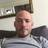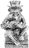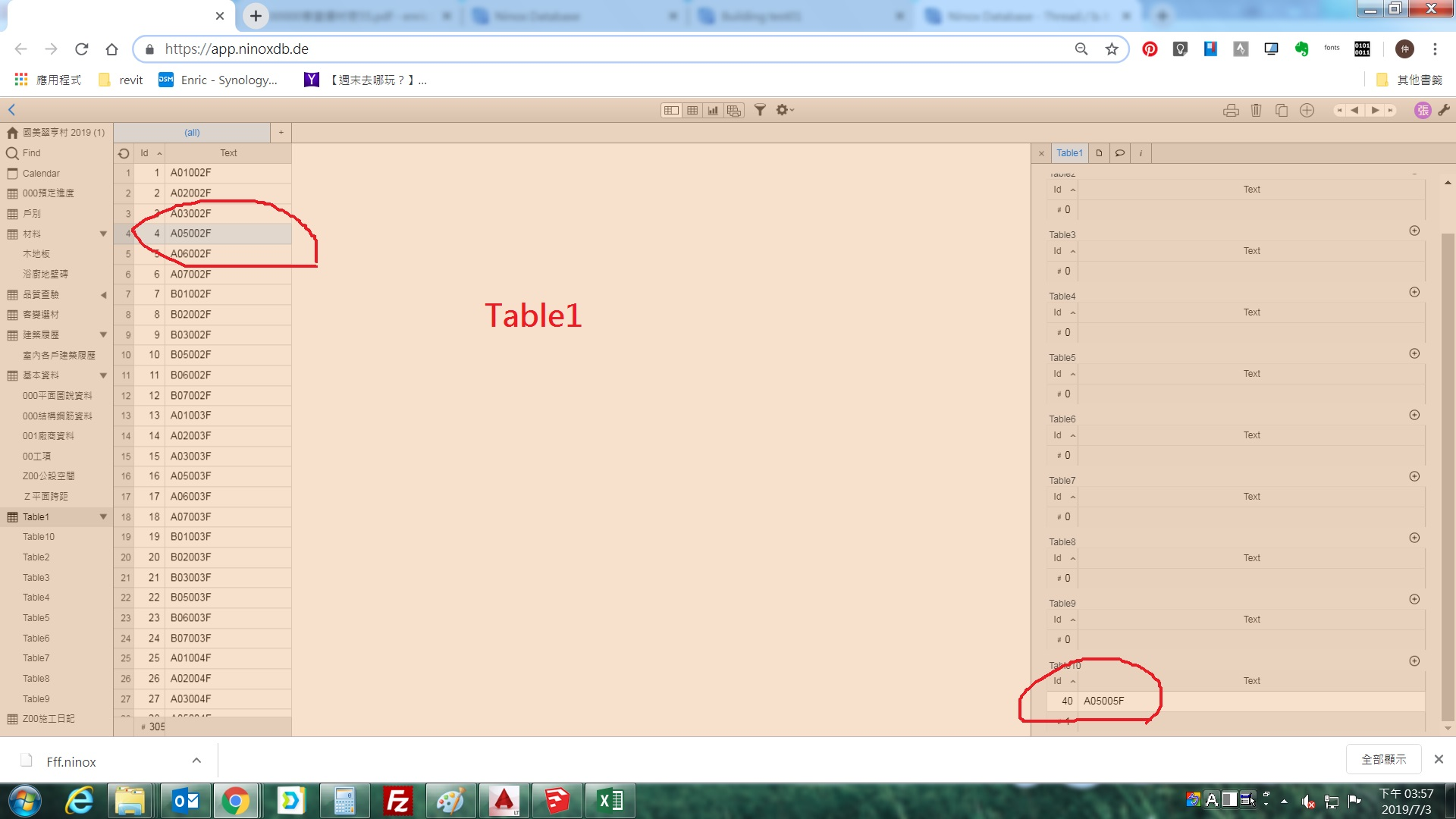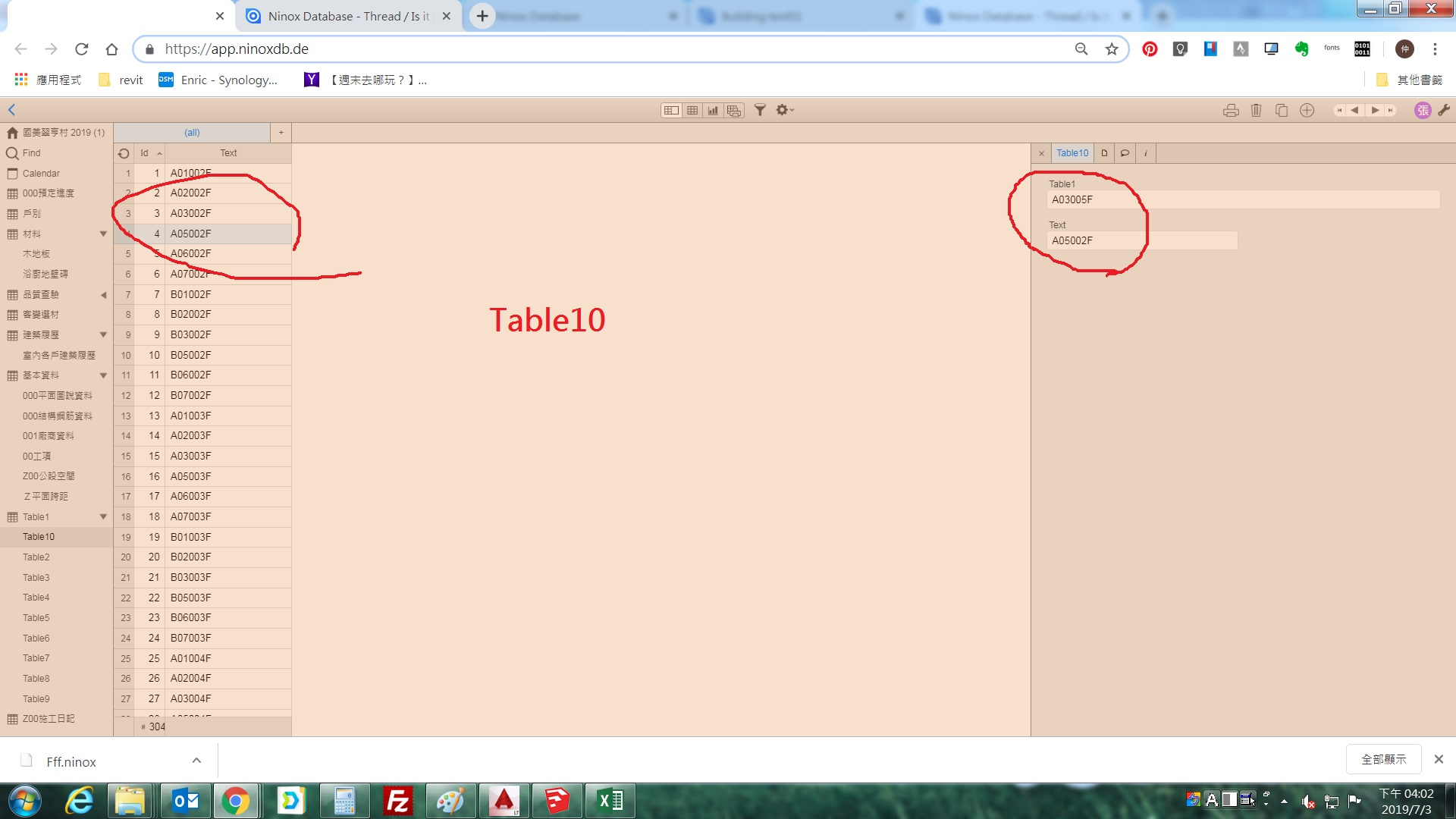### Is it possible to link automatically (via a function) fields in two tables that contain the same text?Wunderbarr !! Thanks ;)You're welcome. I'm glad you got it working Pierre. :)

I would like to ask more than two tables, what should I do? thank you.Have you tried linking the tables one at a time?

Excuses me, I don't understand this. If there is an image description or coding method, I am a newcomer to coding.From the 4th post in this thread...

`let myTable1Id := (select Table1).Id;`
`let myTable1Text := (select Table1).Text;`
`myTable1Text := unique(myTable1Text);`
`let myTable1Cnt := count(myTable1Id);`
`for i in range(0, myTable1Cnt) do`
`for j in (select Table2)[Text = item(myTable1Text, i)] do`
`j.(Table1 := item(myTable1Id, i))`
`end`
`end`

Without information like table names and field names I can't really do much more than give you the generic code above which you would attach to a button. Table1 is the "1" table and Table2 is the "N" table.Additionally, you would replace the generic names Table1 and Table2 with your respective table names. You would also replace "Text" with field names you are using to link the tables.

Thank you , Sean.let myTable1 := Id;
let myText := Text;
for i in (select Table2)[Text = myText] do
i.(Table1 := myTable1)
endlet myTable1Id := (select Table1).Id;
let myTable1Text := (select Table1).Text;
myTable1Text := unique(myTable1Text);
let myTable1Cnt := count(myTable1Id);
for i in range(0, myTable1Cnt) do
for j in (select Table10)[Text = item(myTable1Text, i)] do
j.(Table1 := item(myTable1Id, i))
end
end

Excuse me, What is wrong with this code?(Can't connect the same text)Just to be clear, the first code will only link the records in Table10 where the Text field matches the Text field in Table1 of the currently selected record in Table1.

I'm not sure why it's not working for you. I created a database with Table1 and Table10 and added records A01002F through A06002F and it worked using the code you posted. The only way I can duplicate the results you are getting is the record in Table10 was previously linked to the record in Table1. The code won't update the link if the data changes. You would have to unlink the record and run the code again.

If you have a lot of records that are linked, but don't match you can use this code in a button to unlink all the records...

`for i in select Table10 do`
`i.(Table1 := 0)`
`end`